GeeksforGeeks App
Open AppBrowser
Continue

# Ukkonen’s Suffix Tree Construction – Part 2

In Ukkonen’s Suffix Tree Construction – Part 1, we have seen high level Ukkonen’s Algorithm. This 2nd part is continuation of Part 1.
Please go through Part 1, before looking at current article.

In Suffix Tree Construction of string S of length m, there are m phases and for a phase j (1 <= j <= m), we add jth character in tree built so far and this is done through j extensions. All extensions follow one of the three extension rules (discussed in Part 1).

To do jth extension of phase i+1 (adding character S[i+1]), we first need to find end of the path from the root labelled S[j..i] in the current tree. One way is start from root and traverse the edges matching S[j..i] string. This will take O(m3) time to build the suffix tree. Using few observations and implementation tricks, it can be done in O(m) which we will see now.

For an internal node v with path-label xA, where x denotes a single character and A denotes a (possibly empty) substring, if there is another node s(v) with path-label A, then a pointer from v to s(v) is called a suffix link.
If A is empty string, suffix link from internal node will go to root node.
There will not be any suffix link from root node (As it’s not considered as internal node).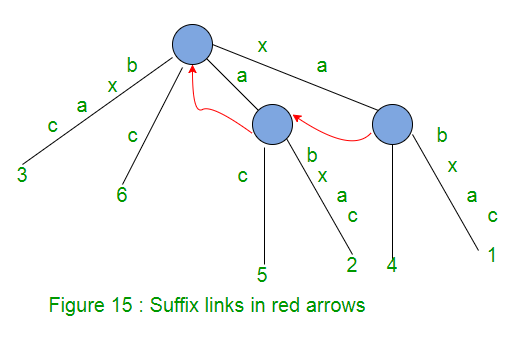In extension j of some phase i, if a new internal node v with path-label xA is added, then in extension j+1 in the same phase i:

• Either the path labelled A already ends at an internal node (or root node if A is empty)
• OR a new internal node at the end of string A will be created

In extension j+1 of same phase i, we will create a suffix link from the internal node created in jth extension to the node with path labelled A.

So in a given phase, any newly created internal node (with path-label xA) will have a suffix link from it (pointing to another node with path-label A) by the end of the next extension.

In any implicit suffix tree Ti after phase i, if internal node v has path-label xA, then there is a node s(v) in Ti with path-label A and node v will point to node s(v) using suffix link.

At any time, all internal nodes in the changing tree will have suffix links from them to another internal node (or root) except for the most recently added internal node, which will receive its suffix link by the end of the next extension.

How suffix links are used to speed up the implementation?
In extension j of phase i+1, we need to find the end of the path from the root labelled S[j..i] in the current tree. One way is start from root and traverse the edges matching S[j..i] string. Suffix links provide a short cut to find end of the path.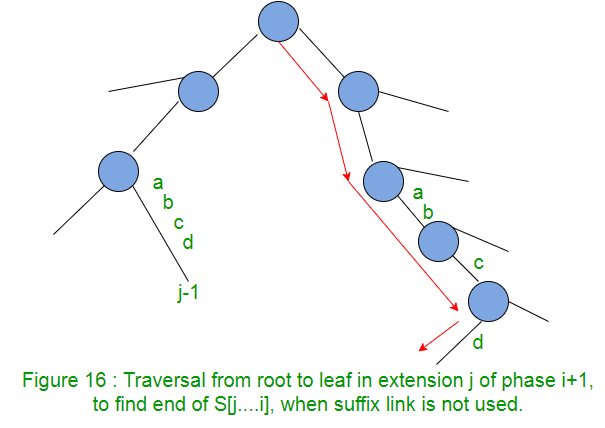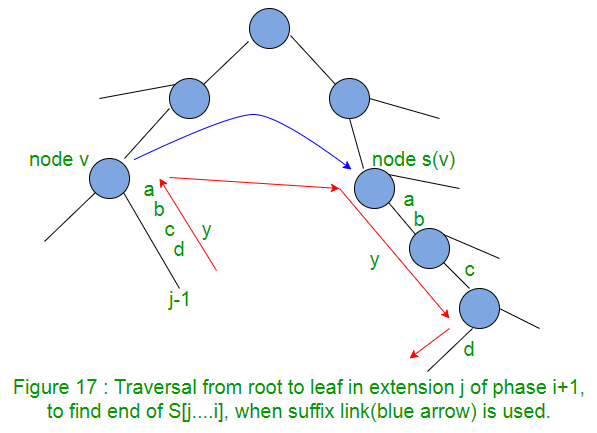So we can see that, to find end of path S[j..i], we need not traverse from root. We can start from the end of path S[j-1..i], walk up one edge to node v (i.e. go to parent node), follow the suffix link to s(v), then walk down the path y (which is abcd here in Figure 17).
This shows the use of suffix link is an improvement over the process.
Note: In the next part 3, we will introduce activePoint which will help to avoid “walk up”. We can directly go to node s(v) from node v.

When there is a suffix link from node v to node s(v), then if there is a path labelled with string y from node v to a leaf, then there must be a path labelled with string y from node s(v) to a leaf. In Figure 17, there is a path label “abcd” from node v to a leaf, then there is a path will same label “abcd” from node s(v) to a leaf.
This fact can be used to improve the walk from s(v) to leaf along the path y. This is called “skip/count” trick.

Skip/Count Trick
When walking down from node s(v) to leaf, instead of matching path character by character as we travel, we can directly skip to the next node if number of characters on the edge is less than the number of characters we need to travel. If number of characters on the edge is more than the number of characters we need to travel, we directly skip to the last character on that edge.
If implementation is such a way that number of characters on any edge, character at a given position in string S should be obtained in constant time, then skip/count trick will do the walk down in proportional to the number of nodes on it rather than the number of characters on it.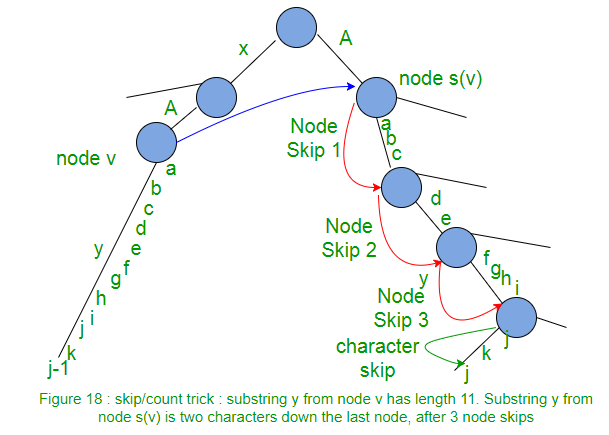Using suffix link along with skip/count trick, suffix tree can be built in O(m2) as there are m phases and each phase takes O(m).

Edge-label compression
So far, path labels are represented as characters in string. Such a suffix tree will take O(m2) space to store the path labels. To avoid this, we can use two pair of indices (start, end) on each edge for path labels, instead of substring itself. The indices start and end tells the path label start and end position in string S. With this, suffix tree needs O(m) space.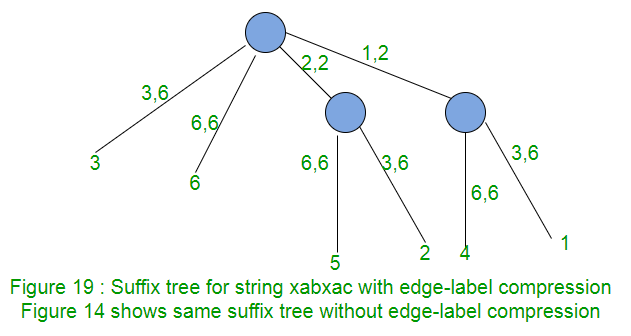There are two observations about the way extension rules interact in successive extensions and phases. These two observations lead to two more implementation tricks (first trick “skip/count” is seen already while walk down).

Observation 1: Rule 3 is show stopper
In a phase i, there are i extensions (1 to i) to be done.
When rule 3 applies in any extension j of phase i+1 (i.e. path labelled S[j..i] continues with character S[i+1]), then it will also apply in all further extensions of same phase (i.e. extensions j+1 to i+1 in phase i+1). That’s because if path labelled S[j..i] continues with character S[i+1], then path labelled S[j+1..i], S[j+2..i], S[j+3..i],…, S[i..i] will also continue with character S[i+1].
Consider Figure 11, Figure12 and Figure 13 in Part 1 where Rule 3 is applied.
In Figure 11, “xab” is added in tree and in Figure 12 (Phase 4), we add next character “x”. In this, 3 extensions are done (which adds 3 suffixes). Last suffix “x” is already present in tree.
In Figure 13, we add character “a” in tree (Phase 5). First 3 suffixes are added in tree and last two suffixes “xa” and “a” are already present in tree. This shows that if suffix S[j..i] present in tree, then ALL the remaining suffixes S[j+1..i], S[j+2..i], S[j+3..i],…, S[i..i] will also be there in tree and no work needed to add those remaining suffixes.
So no more work needed to be done in any phase as soon as rule 3 applies in any extension in that phase. If a new internal node v gets created in extension j and rule 3 applies in next extension j+1, then we need to add suffix link from node v to current node (if we are on internal node) or root node. ActiveNode, which will be discussed in part 3, will help while setting suffix links.

Trick 2
Stop the processing of any phase as soon as rule 3 applies. All further extensions are already present in tree implicitly.

Observation 2: Once a leaf, always a leaf
Once a leaf is created and labelled j (for suffix starting at position j in string S), then this leaf will always be a leaf in successive phases and extensions. Once a leaf is labelled as j, extension rule 1 will always apply to extension j in all successive phases.
Consider Figure 9 to Figure 14 in Part 1.
In Figure 10 (Phase 2), Rule 1 is applied on leaf labelled 1. After this, in all successive phases, rule 1 is always applied on this leaf.
In Figure 11 (Phase 3), Rule 1 is applied on leaf labelled 2. After this, in all successive phases, rule 1 is always applied on this leaf.
In Figure 12 (Phase 4), Rule 1 is applied on leaf labelled 3. After this, in all successive phases, rule 1 is always applied on this leaf.

In any phase i, there is an initial sequence of consecutive extensions where rule 1 or rule 2 are applied and then as soon as rule 3 is applied, phase i ends.
Also rule 2 creates a new leaf always (and internal node sometimes).
If Ji represents the last extension in phase i when rule 1 or 2 was applied (i.e after ith phase, there will be Ji leaves labelled 1, 2, 3, …, Ji) , then Ji <= Ji+1
Ji will be equal to Ji+1 when there are no new leaf created in phase i+1 (i.e rule 3 is applied in Ji+1 extension)
In Figure 11 (Phase 3), Rule 1 is applied in 1st two extensions and Rule 2 is applied in 3rd extension, so here J3 = 3
In Figure 12 (Phase 4), no new leaf created (Rule 1 is applied in 1st 3 extensions and then rule 3 is applied in 4th extension which ends the phase). Here J4 = 3 = J3
In Figure 13 (Phase 5), no new leaf created (Rule 1 is applied in 1st 3 extensions and then rule 3 is applied in 4th extension which ends the phase). Here J5 = 3 = J4
Ji will be less than Ji+1 when few new leaves are created in phase i+1.
In Figure 14 (Phase 6), new leaf created (Rule 1 is applied in 1st 3 extensions and then rule 2 is applied in last 3 extension which ends the phase). Here J6 = 6 > J5

So we can see that in phase i+1, only rule 1 will apply in extensions 1 to Ji (which really doesn’t need much work, can be done in constant time and that’s the trick 3), extension Ji+1 onwards, rule 2 may apply to zero or more extensions and then finally rule 3, which ends the phase.
Now edge labels are represented using two indices (start, end), for any leaf edge, end will always be equal to phase number i.e. for phase i, end = i for leaf edges, for phase i+1, end = i+1 for leaf edges.

Trick 3
In any phase i, leaf edges may look like (p, i), (q, i), (r, i), …. where p, q, r are starting position of different edges and i is end position of all. Then in phase i+1, these leaf edges will look like (p, i+1), (q, i+1), (r, i+1),…. This way, in each phase, end position has to be incremented in all leaf edges. For this, we need to traverse through all leaf edges and increment end position for them. To do same thing in constant time, maintain a global index e and e will be equal to phase number. So now leaf edges will look like (p, e), (q, e), (r, e).. In any phase, just increment e and extension on all leaf edges will be done. Figure 19 shows this.

So using suffix links and tricks 1, 2 and 3, a suffix tree can be built in linear time.

Tree Tm could be implicit tree if a suffix is prefix of another. So we can add a \$ terminal symbol first and then run algorithm to get a true suffix tree (A true suffix tree contains all suffixes explicitly). To label each leaf with corresponding suffix starting position (all leaves are labelled as global index e), a linear time traversal can be done on tree.

At this point, we have gone through most of the things we needed to know to create suffix tree using Ukkonen’s algorithm. In next Part 3, we will take string S = “abcabxabcd” as an example and go through all the things step by step and create the tree. While building the tree, we will discuss few more implementation issues which will be addressed by ActivePoints.
We will continue to discuss the algorithm in Part 4 and Part 5. Code implementation will be discussed in Part 6.

References:
http://web.stanford.edu/~mjkay/gusfield.pdf